NCERT Exemplar - MCQs

Chapter 5 Class 12 Continuity and Differentiability
Serial order wise

## (D) 1.5

This question is similar to Example 15 - Chapter 5 Class 12 Continuity and Differentiability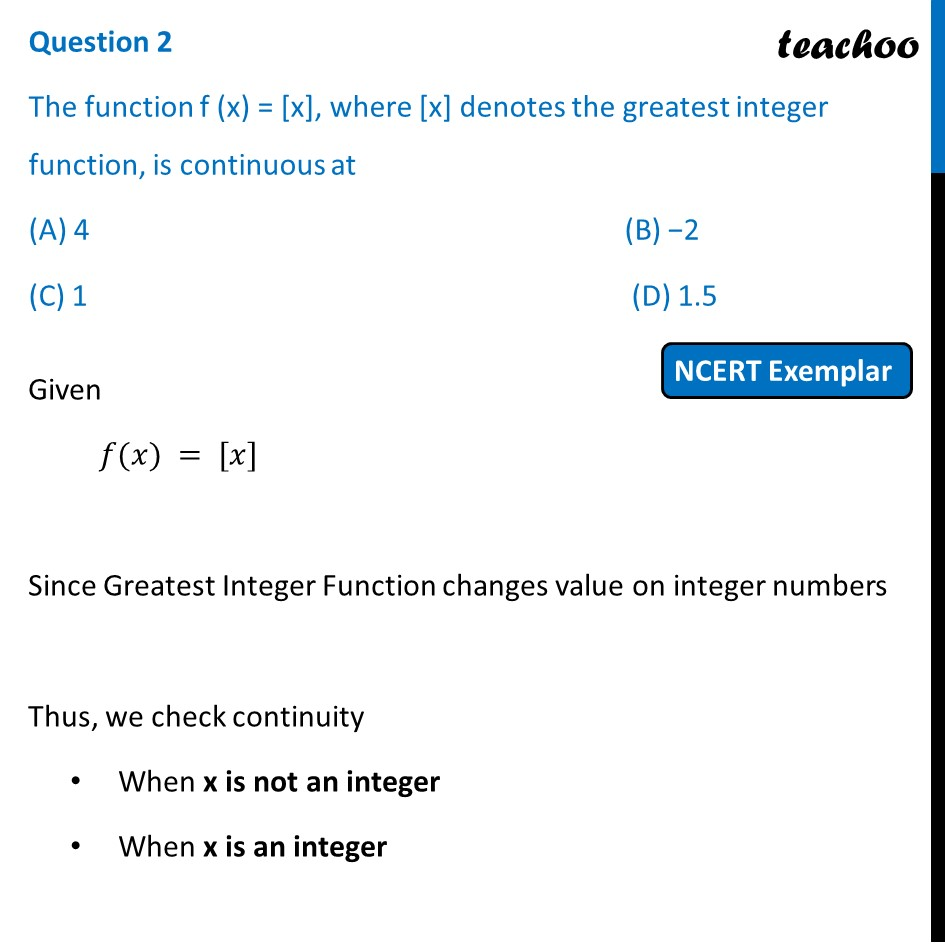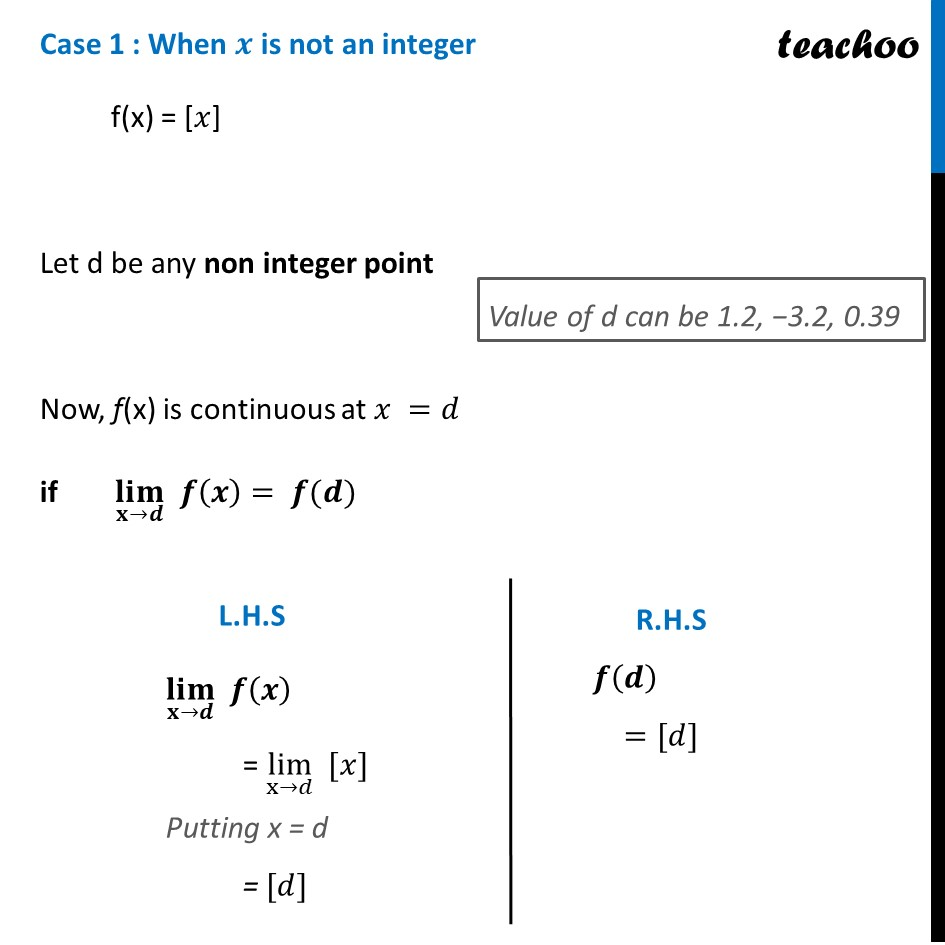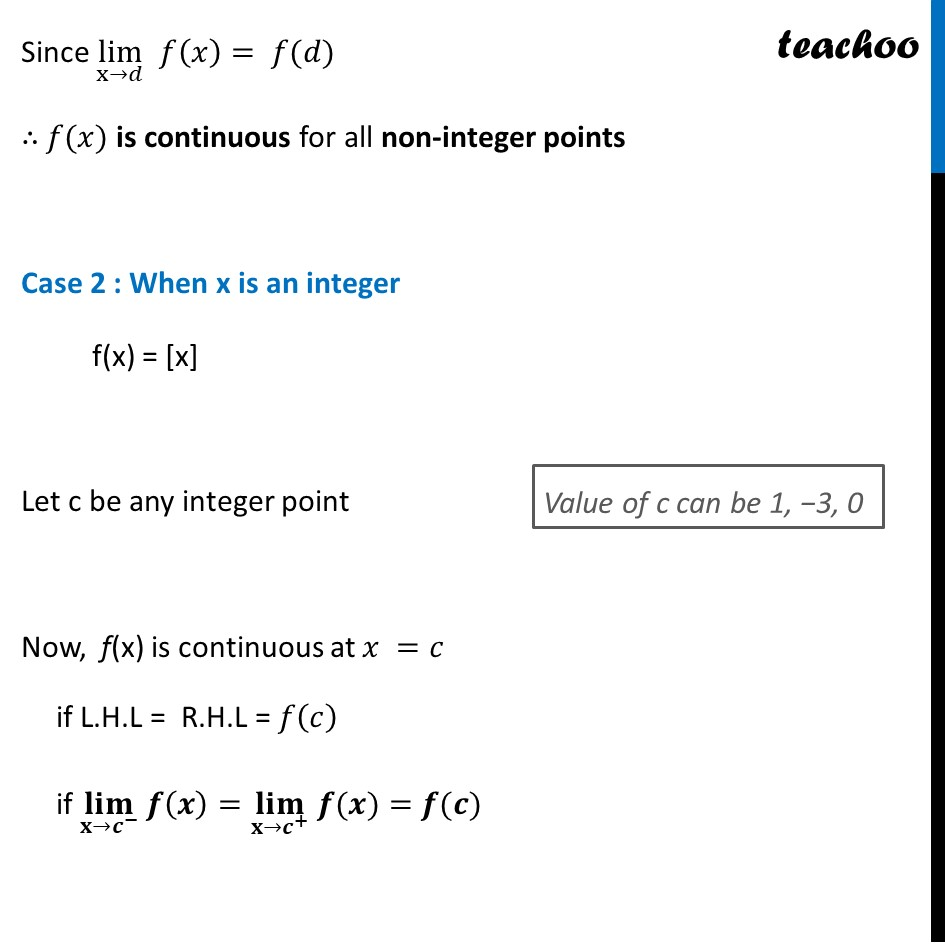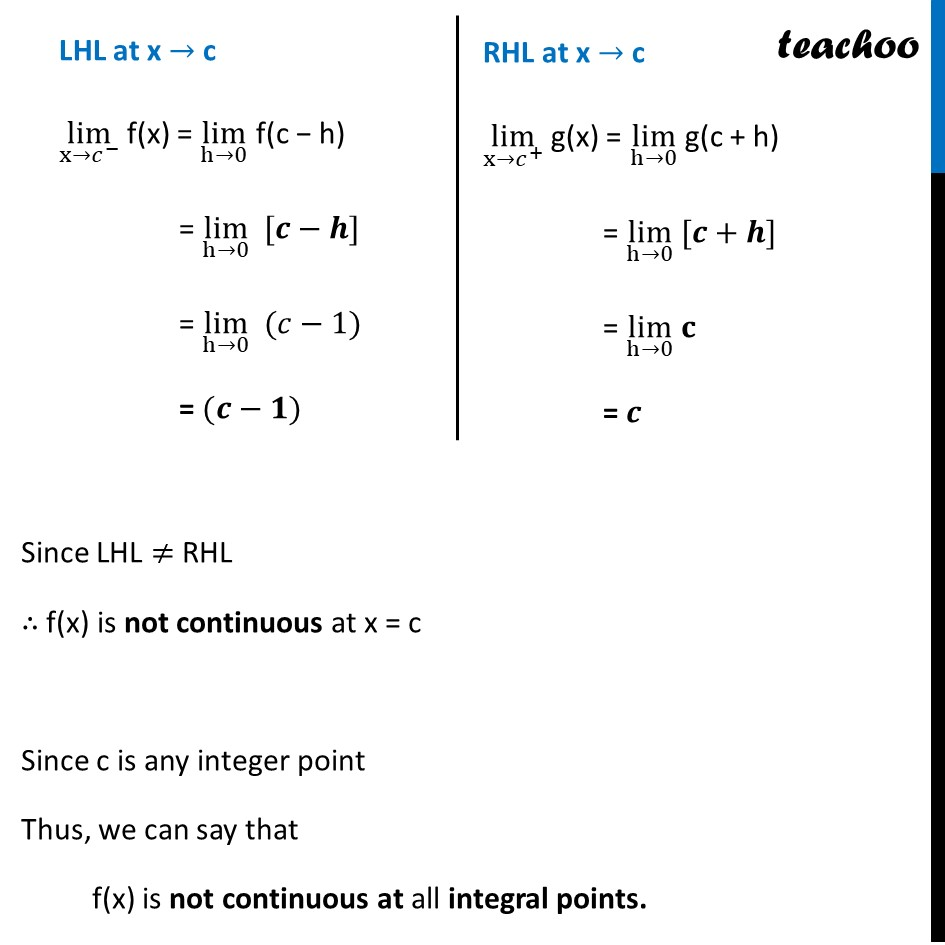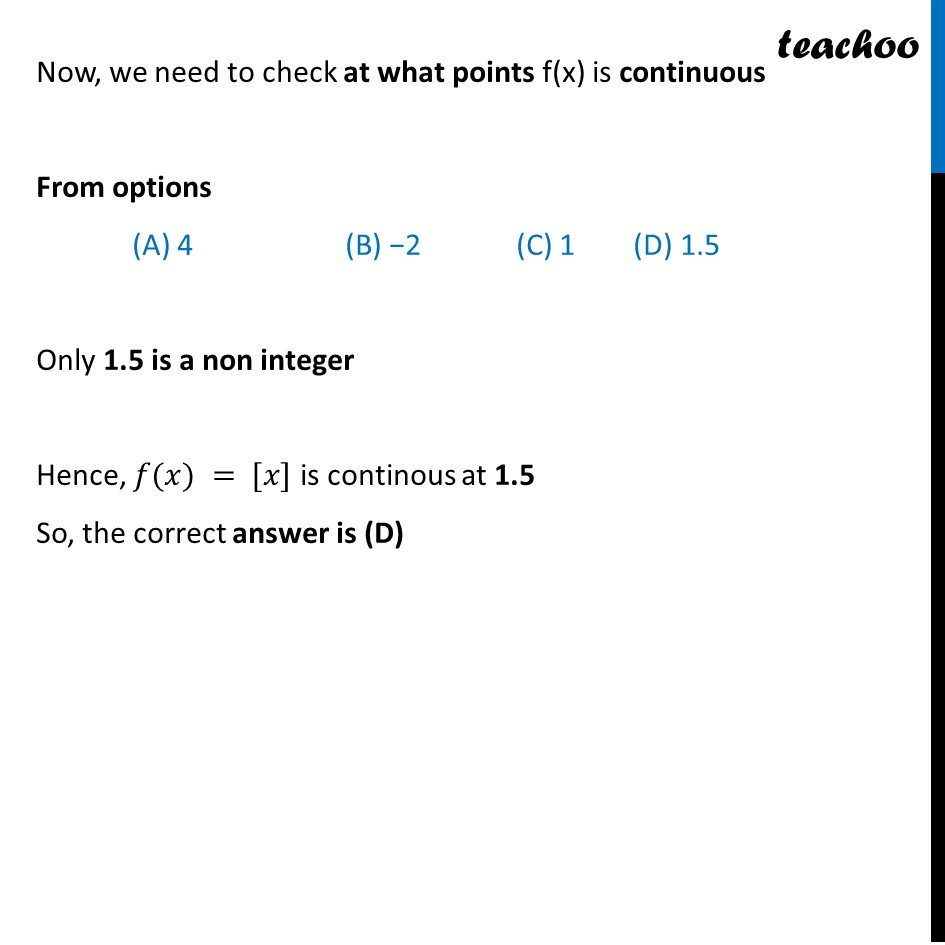Learn in your speed, with individual attention - Teachoo Maths 1-on-1 Class

### Transcript

Question 2 The function f (x) = [x], where [x] denotes the greatest integer function, is continuous at (A) 4 (B) −2 (C) 1 (D) 1.5 Given 𝑓(𝑥) = [𝑥] Since Greatest Integer Function changes value on integer numbers Thus, we check continuity When x is not an integer When x is an integer Case 1 : When 𝒙 is not an integer f(x) = [𝑥] Let d be any non integer point Now, f(x) is continuous at 𝑥 =𝑑 if (𝐥𝐢𝐦)┬(𝐱→𝒅) 𝒇(𝒙)= 𝒇(𝒅) (𝐥𝐢𝐦)┬(𝐱→𝒅) 𝒇(𝒙) = lim┬(x→𝑑) [𝑥] Putting x = d = [𝑑] 𝒇(𝒅) =[𝑑] Since lim┬(x→𝑑) 𝑓(𝑥)= 𝑓(𝑑) ∴ 𝑓(𝑥) is continuous for all non-integer points Case 2 : When x is an integer f(x) = [x] Let c be any integer point Now, f(x) is continuous at 𝑥 =𝑐 if L.H.L = R.H.L = 𝑓(𝑐) if (𝐥𝐢𝐦)┬(𝐱→𝒄^− ) 𝒇(𝒙)=(𝐥𝐢𝐦)┬(𝐱→𝒄^+ ) " " 𝒇(𝒙)=𝒇(𝒄) LHL at x → c lim┬(x→𝑐^− ) f(x) = lim┬(h→0) f(c − h) = lim┬(h→0) [𝒄−𝒉] = lim┬(h→0) (𝑐−1) = (𝒄−𝟏) RHL at x → c lim┬(x→𝑐^+ ) g(x) = lim┬(h→0) g(c + h) = lim┬(h→0) [𝒄+𝒉] = lim┬(h→0) 𝐜 = 𝒄 Now, we need to check at what points f(x) is continuous From options (A) 4 (B) −2 (C) 1 (D) 1.5 Only 1.5 is a non integer Hence, 𝑓(𝑥) = [𝑥] is continous at 1.5 So, the correct answer is (D)